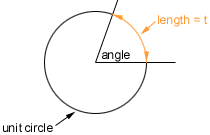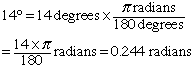Name: Amy Who is asking: Student Level: Secondary Question: i have to find out what is meant by the radian measure of an angle and compare it to the measure of an angle in degrees. Thanks! Hi Amy, If you have an angle you can measure its size in the following way. Draw a unit circle (circle with radius 1) with center at the vertex of the angle.Measure the length of the arc (counterclockwise) subtended by the angle (the length of the orange arc in the diagram). Suppose that this length is t, then we say that the radian measure of the angle is t radians. Since the circumference of the unit circle is 2 pi, the 360o angle has radian measure of 2 pi. Likewise 180o is pi radians (half way around the circle). 90o is  pi/2 radians and 45o is  pi/4. For other angles I remember that 180o = pi radians Thus, for example,One last point is that if you measure the arc clockwise then the radian measure is negative. Thus -45o is  -pi/4 radians. Cheers, Harley Go to Math Central